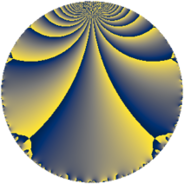Properties

 Label 42.3.hLevel $42$ Weight $3$ Character orbit 42.h Rep. character $\chi_{42}(11,\cdot)$ Character field $\Q(\zeta_{6})$ Dimension $12$ Newform subspaces $2$ Sturm bound $24$ Trace bound $1$

Related objects

Defining parameters

 Level: $$N$$ $$=$$ $$42 = 2 \cdot 3 \cdot 7$$ Weight: $$k$$ $$=$$ $$3$$ Character orbit: $$[\chi]$$ $$=$$ 42.h (of order $$6$$ and degree $$2$$) Character conductor: $$\operatorname{cond}(\chi)$$ $$=$$ $$21$$ Character field: $$\Q(\zeta_{6})$$ Newform subspaces: $$2$$ Sturm bound: $$24$$ Trace bound: $$1$$ Distinguishing $$T_p$$: $$5$$

Dimensions

The following table gives the dimensions of various subspaces of $$M_{3}(42, [\chi])$$.

Total New Old
Modular forms 40 12 28
Cusp forms 24 12 12
Eisenstein series 16 0 16

Trace form

 $$12 q + 12 q^{4} + 8 q^{6} - 14 q^{7} + 4 q^{9} + O(q^{10})$$ $$12 q + 12 q^{4} + 8 q^{6} - 14 q^{7} + 4 q^{9} - 8 q^{10} - 68 q^{13} - 68 q^{15} - 24 q^{16} - 24 q^{18} + 66 q^{19} - 2 q^{21} - 16 q^{22} + 8 q^{24} + 70 q^{25} + 252 q^{27} + 16 q^{28} + 76 q^{30} + 34 q^{31} - 106 q^{33} - 16 q^{34} + 16 q^{36} + 6 q^{37} - 74 q^{39} + 16 q^{40} - 144 q^{42} + 84 q^{43} - 154 q^{45} + 80 q^{46} - 138 q^{49} - 34 q^{51} - 68 q^{52} + 8 q^{54} - 472 q^{55} - 144 q^{57} - 128 q^{58} - 68 q^{60} + 112 q^{61} + 276 q^{63} - 96 q^{64} + 224 q^{66} + 26 q^{67} + 260 q^{69} + 392 q^{70} + 48 q^{72} + 326 q^{73} + 70 q^{75} + 264 q^{76} + 224 q^{78} + 154 q^{79} + 64 q^{81} - 48 q^{82} - 152 q^{84} + 440 q^{85} - 164 q^{87} - 16 q^{88} - 592 q^{90} - 338 q^{91} - 224 q^{93} - 480 q^{94} - 16 q^{96} - 88 q^{97} - 140 q^{99} + O(q^{100})$$

Decomposition of $$S_{3}^{\mathrm{new}}(42, [\chi])$$ into newform subspaces

Label Dim. $$A$$ Field CM Traces $q$-expansion
$a_{2}$ $a_{3}$ $a_{5}$ $a_{7}$
42.3.h.a $4$ $1.144$ $$\Q(\sqrt{-2}, \sqrt{-3})$$ None $$0$$ $$2$$ $$0$$ $$-14$$ $$q+\beta _{1}q^{2}+(2\beta _{1}+\beta _{2}-2\beta _{3})q^{3}+2\beta _{2}q^{4}+\cdots$$
42.3.h.b $8$ $1.144$ 8.0.4857532416.2 None $$0$$ $$-2$$ $$0$$ $$0$$ $$q+(\beta _{2}-\beta _{6})q^{2}+(\beta _{1}-\beta _{2}-\beta _{3}+\beta _{4}+\cdots)q^{3}+\cdots$$

Decomposition of $$S_{3}^{\mathrm{old}}(42, [\chi])$$ into lower level spaces

$$S_{3}^{\mathrm{old}}(42, [\chi]) \cong$$ $$S_{3}^{\mathrm{new}}(21, [\chi])$$$$^{\oplus 2}$$